Refer to our Texas Go Math Grade 5 Answer Key Pdf to score good marks in the exams. Test yourself by practicing the problems from Texas Go Math Grade 5 Lesson 5.1 Answer Key Addition with Unequal Denominators.

Investigate

Hilary is using red fabric to make a tote bag. She uses one piece that is $$\frac{1}{2}$$ yard long. She uses another piece that is $$\frac{1}{4}$$ yard long. How much red fabric does she use?

Materials; fraction strips; MathBoard

A. Find $$\frac{1}{2}$$ + $$\frac{1}{4}$$. Place a $$\frac{1}{2}$$ strip and a $$\frac{1}{4}$$ strip under the 1-whole strip on your MathBoard.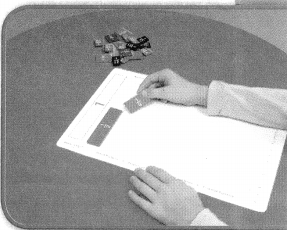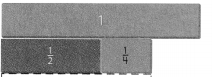B. Find fraction strips, all with the same denominator, that are equivalent to $$\frac{1}{2}$$ and $$\frac{1}{4}$$ and fit exactly under the sum $$\frac{1}{2}$$ + $$\frac{1}{4}$$. Record the addends, using equal denominators.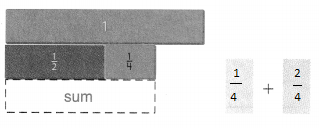C. Record the sum in simplest form. $$\frac{1}{2}$$ + $$\frac{1}{4}$$ = ___________
So, Hilary uses ___________ yard of fabric.
Answer: So, Hilary uses $$\frac{3}{4}$$ yard of fabric.
Record the sum in simplest form. $$\frac{1}{2}$$ + $$\frac{1}{4}$$ = $$\frac{3}{4}$$

Math Talk
Mathematical Processes

How can you tell if the sum of the fractions is less than 1?
Fractions greater than 1 have numerators larger than their denominators; those that are less than 1 have numerators smaller than their denominators; the rest are equal to 1.

Draw Conclusions

Question 1.
Describe how you would determine what fraction strips, all with the same denominator, would fit exactly under $$\frac{1}{2}$$ + $$\frac{1}{3}$$. What are they?Explanation:
The denominator is 6
Which would fit exactly under $$\frac{1}{6}$$ + $$\frac{1}{6}$$+ $$\frac{1}{6}$$ + $$\frac{1}{6}$$+$$\frac{1}{6}$$ + $$\frac{1}{6}$$.

H.O.T. Explain the difference between finding fraction strips with the same denominator for $$\frac{1}{2}$$ + $$\frac{1}{3}$$ and $$\frac{1}{2}$$ + $$\frac{1}{4}$$.

Make Connections

Sometimes, the sum of two fractions is greater than 1. When adding fractions with unequal denominators, you can use the 1-whole strip to help determine If a sum is greater than 1 or less than 1.

Use fraction strips to solve. $$\frac{3}{5}$$ + $$\frac{1}{2}$$
STEP 1:
Work with another student. Place three $$\frac{1}{5}$$ fraction strips under the 1-whole strip on your MathBoard. Then place a $$\frac{1}{2}$$ fraction strip beside the three $$\frac{1}{5}$$ strips.

STEP 2:
Find fraction strips, all with the same denominator, that are equivalent to $$\frac{3}{5}$$ and $$\frac{1}{2}$$. Place the fraction strips under the sum. At the right, draw a picture of the model and write the equivalent fractions.
$$\frac{3}{5}$$ = __________ $$\frac{1}{2}$$ = __________

STEP 3:
Add the fractions with equal denominators. Use the 1-whole strip to rename the sum in simplest form.
$$\frac{3}{5}$$ + $$\frac{1}{2}$$ = __________ + _________
= __________ or _________
Think: How many fraction strips with the same denominator are equal to 1 whole?
step 1: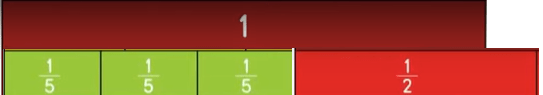Explanation:
Placed three $$\frac{1}{5}$$ fraction strips under the 1-whole strip on your MathBoard. Then place a $$\frac{1}{2}$$ fraction strip beside the three $$\frac{1}{5}$$ strips.
step 2:
Place the fraction strips under the sum. At the right, draw a picture of the model and write the equivalent fractions.
$$\frac{3}{5}$$ = 6$$\frac{1}{10}$$
$$\frac{1}{2}$$ = 5$$\frac{1}{10}$$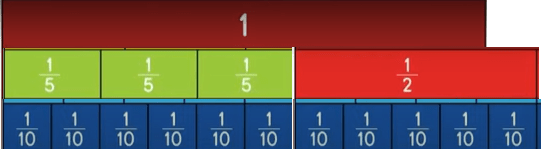step 3:
$$\frac{3}{5}$$ + $$\frac{1}{2}$$ = 6$$\frac {1}{10}$$ + 5$$\frac{1}{10}$$
= $$\frac{11}{10}$$Math Talk
Mathematical Processes

In what step did you find out that the answer is greater than 1? Explain.
Explanation:
$$\frac{3}{5}$$ =6$$\frac{1}{10}$$
$$\frac{1}{2}$$ =5$$\frac{1}{10}$$Share and Show

Use fraction strips to find the sum. Write your answer in simplest form.

Question 1.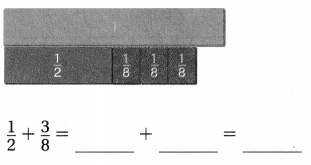Explanation:
Place the fraction strips with the same denominator 8, that are equivalent to $$\frac{1}{2}$$

Go Math Grade 5 Chapter 5 Answer Key Pdf Lesson 5.1 Question 2.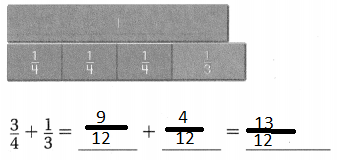Explanation:
Place the fraction strips with the same denominator of 12, that are equivalent to $$\frac{3}{4}$$ and $$\frac{1}{3}$$. Then Added.

Use fraction strips to find the sum. Write your answer in simplest form.

Question 3.
$$\frac{2}{5}$$ + $$\frac{3}{10}$$ = __________
Answer: $$\frac{7}{10}$$
Explanation:
$$\frac{2}{5}$$ + $$\frac{3}{10}$$ = $$\frac{4}{10}$$ + $$\frac{3}{10}$$ = $$\frac{7}{10}$$
orQuestion 4.
$$\frac{1}{4}$$ + $$\frac{1}{12}$$ = ___________
Answer: $$\frac{1}{3}$$
Explanation:
$$\frac{1}{4}$$ + $$\frac{1}{12}$$ = $$\frac{3}{12}$$ + $$\frac{1}{12}$$ = $$\frac{4}{12}$$ = $$\frac{1}{3}$$
or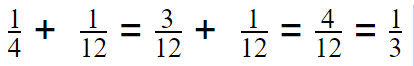Question 5.
$$\frac{1}{2}$$ + $$\frac{3}{10}$$ = ____________
Answer: $$\frac{4}{5}$$
Explanation:
$$\frac{1}{2}$$ + $$\frac{3}{10}$$ = $$\frac{5}{10}$$ + $$\frac{3}{10}$$ = $$\frac{8}{10}$$ = $$\frac{4}{5}$$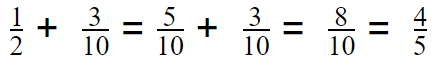Problem Solving

Question 6.
H.O.T. Multi-Step Maya makes trail mix by combining $$\frac{1}{3}$$ cup mixed nuts, $$\frac{1}{4}$$ cup of dried fruit, and $$\frac{1}{6}$$ cup of chocolate morsels. What is the total amount of ingredients in her trail mix?Answer: $$\frac{3}{4}$$
Explanation:
$$\frac{1}{3}$$ + $$\frac{1}{4}$$ + $$\frac{1}{6}$$= $$\frac{4}{12}$$ + $$\frac{3}{12}$$ +$$\frac{2}{12}$$ = $$\frac{9}{12}$$ = $$\frac{3}{4}$$

Go Math Practice and Homework Lesson 5.1 Answer Key Question 7.
H.O.T. Pose a Problem Write a new problem using different amounts of ingredients Maya used. Each amount should be a fraction with a denominator of 2, 3, or 4.
Maya makes trail mix by combining $$\frac{1}{2}$$ cup mixed nuts, $$\frac{1}{3}$$ cup of dried fruit, and $$\frac{1}{4}$$ cup of chocolate morsels. What is the total amount of ingredients in her trail mix?

Question 8.
Use Diagrams to Solve the problem you wrote. Draw a picture of the fraction strips you use to solve your problem.
Answer: $$\frac{13}{12}$$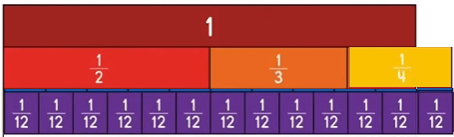Question 9.
Explain why you chose the amounts you did for your problem.
Answer: in the question asked use different amounts for ingredients and Each amount should be a fraction with a denominator of 2, 3, or 4.

Question 10.
Write Math Explain how using fraction strips with equal denominators makes it possible to add fractions with unequal denominators.

Think of the fruit analogy?

Does it make sense to add two bananas plus one watermelon? The units do not make sense for the sum.

BUT … we could think about changing both of them to common units, servings of fruit. If one banana serves one person and one watermelon serves ten people, then we could convert:

• two bananas + one watermelon =
• = two fruit servings + ten fruit servings =
• = twelve servings of fruit

Example:Question 11.
In a garden, bluebonnets occupy $$\frac{7}{10}$$ of the garden. After winter, the bluebonnets spread to cover another $$\frac{1}{5}$$ of the garden. What fraction of the garden is now covered in bluebonnets?
(A) $$\frac{1}{5}$$
(B) $$\frac{1}{2}$$
(C) $$\frac{8}{10}$$
(D) $$\frac{9}{10}$$
Explanation:
$$\frac{7}{10}$$ + $$\frac{1}{5}$$  = $$\frac{9}{10}$$Question 12.
Ling is using fraction strips to add $$\frac{2}{3}$$ and $$\frac{7}{12}$$. The sum is one whole, plus how many twelfths?
(A) 1
(B) 2
(C) 3
(D) 4
Explanation: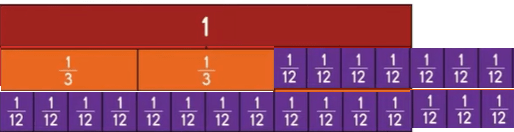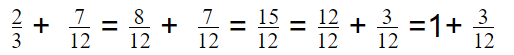Question 13.
Multi-Step Juan uses $$\frac{1}{5}$$ liter to water a small plant, and he uses $$\frac{1}{2}$$ liter to water a large plant. Now he has $$\frac{2}{10}$$ liter Left in the pitcher. How much water did Juan have in the beginning?
(A) $$\frac{3}{5}$$ L
(B) $$\frac{9}{10}$$ L
(C) $$\frac{1}{10}$$ L
(D) $$\frac{3}{10}$$ L
Explanation:
Juan uses $$\frac{1}{5}$$ liter to water a small plant, and he uses $$\frac{1}{2}$$ liter to water a large plant.
So total water he used $$\frac{7}{10}$$ L.
Now he has $$\frac{2}{10}$$ liter Left in the pitcher.
So Juan have water in the beginning is $$\frac{9}{10}$$ L. Since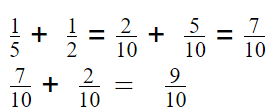Texas Test Prep

Wilhelm is making a pie. He uses $$\frac{1}{2}$$ cup of blueberries and $$\frac{2}{3}$$ cup of raspberries. What is the total amount of berries in Wilhelm’s pie?
(A) $$\frac{3}{5}$$ cup
(B) $$\frac{2}{6}$$ cup
(C) $$\frac{7}{6}$$ cups
(D) $$\frac{3}{6}$$ cup
Answer: $$\frac{7}{6}$$ cups
Explanation:
He uses $$\frac{1}{2}$$ cup of blueberries and $$\frac{2}{3}$$ cup of raspberries.
Sum of  $$\frac{1}{2}$$  + $$\frac{2}{3}$$ = $$\frac{7}{6}$$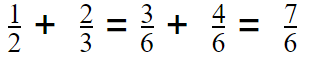### Texas Go Math Grade 5 Lesson 5.1 Homework and Practice Answer Key

Use fraction strips to find the sum. Write your answer in the simplest form.

Question 1.Explanation:
To add the given fractions, did the denominator equal first then added

Question 2.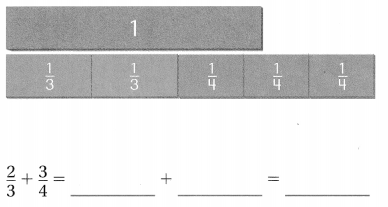Explanation:
To add the given fractions, did the denominator equal first then added

Question 3.
$$\frac{1}{6}$$ + $$\frac{3}{4}$$ = ____________
Answer:$$\frac{11}{12}$$
Explanation:
To add the given fractions, did the denominator equal first then addedQuestion 4.
$$\frac{5}{6}$$ + $$\frac{1}{2}$$ = ____________
Answer: $$\frac{8}{6}$$
Explanation:
To add the given fractions, did the denominator equal first then added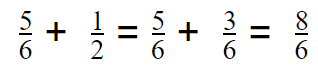Question 5.
$$\frac{1}{2}$$ + $$\frac{2}{5}$$ = _____________
Answer: $$\frac{9}{10}$$
Explanation:
To add the given fractions, did the denominator equal first then added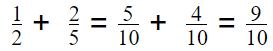Question 6.
$$\frac{1}{4}$$ + $$\frac{2}{3}$$ = ______________
Answer: $$\frac{11}{12}$$
Explanation:
To add the given fractions, did the denominator equal first then added
$$\frac{1}{4}$$ + $$\frac{2}{3}$$ = $$\frac{3}{12}$$ + $$\frac{8}{12}$$ = $$\frac{11}{12}$$

Question 7.
$$\frac{1}{3}$$ + $$\frac{5}{6}$$ = _____________
Answer: $$\frac{7}{6}$$
Explanation:
To add the given fractions, did the denominator equal first then added
$$\frac{1}{3}$$ + $$\frac{5}{6}$$ = $$\frac{2}{6}$$ + $$\frac{5}{6}$$ = $$\frac{7}{6}$$

Question 8.
$$\frac{3}{5}$$ + $$\frac{3}{10}$$ = ______________
Answer: $$\frac{9}{10}$$
Explanation:
To add the given fractions, did the denominator equal first then added
$$\frac{3}{5}$$ + $$\frac{3}{10}$$ = $$\frac{6}{10}$$ + $$\frac{3}{10}$$ = $$\frac{9}{10}$$

Question 9.
$$\frac{1}{8}$$ + $$\frac{3}{4}$$ = _____________
Answer: $$\frac{7}{8}$$
Explanation:
To add the given fractions, did the denominator equal first then added
$$\frac{1}{8}$$ + $$\frac{3}{4}$$ = $$\frac{1}{8}$$ + $$\frac{6}{8}$$ = $$\frac{7}{8}$$

Question 10.
$$\frac{7}{10}$$ + $$\frac{1}{2}$$ = _____________
Answer: $$\frac{7}{8}$$
Explanation:
To add the given fractions, did the denominator equal first then added
$$\frac{7}{10}$$ + $$\frac{1}{2}$$ = $$\frac{7}{10}$$ + $$\frac{5}{10}$$ = $$\frac{12}{10}$$ = $$\frac{6}{5}$$

Question 11.
$$\frac{5}{6}$$ + $$\frac{1}{12}$$ = _____________
Answer: $$\frac{11}{12}$$
Explanation:
To add the given fractions, did the denominator equal first then added
$$\frac{5}{6}$$ + $$\frac{1}{12}$$ = $$\frac{10}{12}$$ + $$\frac{1}{12}$$ = $$\frac{11}{12}$$

Problem Solving

Question 12.
Cooper is grating cheese for the family taco dinner. He grates $$\frac{1}{2}$$ cup of cheddar cheese and $$\frac{3}{4}$$ cup of monterey jack cheese. How much cheese does Cooper grate?
Answer:  Cooper grate $$\frac{5}{4}$$ cup of cheese
Explanation:
Cooper grates $$\frac{1}{2}$$ cup of cheddar cheese and $$\frac{3}{4}$$ cup of monterey jack cheese. then total cheese is sum of $$\frac{1}{2}$$ cup of cheddar cheese and $$\frac{3}{4}$$ cup of monterey jack cheese. So Total cheese is $$\frac{5}{4}$$
$$\frac{1}{2}$$+$$\frac{3}{4}$$  =$$\frac{2}{4}$$+$$\frac{3}{4}$$  = $$\frac{5}{4}$$

Jasmine has to mix $$\frac{3}{4}$$ cup of flour and $$\frac{3}{8}$$ cup of cornmeal. She has a container that holds 1 cup. Can Jasmine mix the flour and cornmeal in the container? Explain.
Answer: Jasmine Can not mix the flour and cornmeal in the container. Since the total mix of flour and cornmeal is 1/8 cup more than a cup.
Explanation:
Jasmine has to mix $$\frac{3}{4}$$ cup of flour and $$\frac{3}{8}$$ cup of cornmeal. So Sum of flour and cornmeal is $$\frac{9}{8}$$
if divided into fraction strips of the same denominator the $$\frac{8}{8}$$ + $$\frac{1}{8}$$. $$\frac{8}{8}$$ is equals to 1 cup. So she can not mix in the container.

Lesson Check

Question 14.
Julio spent $$\frac{1}{10}$$ of his weekly allowance on a set of markers and $$\frac{2}{5}$$ of it on a book. What fraction of Julio’s allowance is this altogether?
(A) $$\frac{1}{2}$$
(B) $$\frac{3}{10}$$
(C) $$\frac{3}{5}$$
(D) $$\frac{1}{5}$$
Answer: $$\frac{1}{2}$$
Explanation:
$$\frac{1}{10}$$ + $$\frac{2}{5}$$  = $$\frac{1}{10}$$ + $$\frac{4}{10}$$ =$$\frac{5}{10}$$ = $$\frac{1}{2}$$

Question 15.
Kate is using fraction strips to add $$\frac{4}{10}$$ and $$\frac{4}{5}$$. She uses one whole strip to represent the sum. How many fifth strips does she need to complete the sum?
(A) 1
(B) 2
(C) 5
(D) 8
Explanation: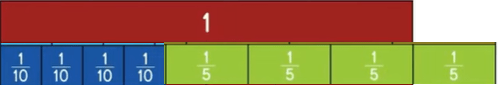one extra strip is needed to complete the sum
Question 16.
Which fraction correctly completes the equation?
$$\frac{6}{8}$$ + $$\frac{}{}$$ = 1
(A) $$\frac{1}{2}$$
(B) $$\frac{1}{8}$$
(C) $$\frac{3}{8}$$
(D) $$\frac{1}{4}$$
Explanation:
$$\frac{6}{8}$$ + $$\frac{1}{4}$$ = $$\frac{6}{8}$$ + $$\frac{2}{8}$$ = $$\frac{8}{8}$$ = 1

Question 17.
An apple was cut into 8 equal-size pieces. Stacy ate $$\frac{1}{4}$$ of the apple. Tony ate $$\frac{3}{8}$$ of the apple. What part of the apple did Stacy and Tony eat in all?
(A) $$\frac{1}{2}$$
(B) $$\frac{5}{8}$$
(C) $$\frac{3}{4}$$
(D) $$\frac{1}{4}$$
Explanation:
Equal the all denominators:
Stacy ate $$\frac{1}{4}$$ of the apple = $$\frac{1}{4}$$ = $$\frac{2}{8}$$
Tony ate $$\frac{3}{8}$$ of the apple = $$\frac{3}{8}$$
Stacy and Tony ate = $$\frac{3}{8}$$  + $$\frac{2}{8}$$= $$\frac{5}{8}$$

Question 18.
Multi-Step Last weekend, Beatrice walked her poodle $$\frac{2}{3}$$ mile on Saturday and $$\frac{5}{6}$$ mile on Sunday. Fiona walked her beagle $$\frac{1}{3}$$ mile on Saturday and $$\frac{1}{2}$$ mile on Sunday. How much farther did the poodle walk last weekend than the beagle?
(A) $$\frac{1}{2}$$ mile
(B) 1$$\frac{1}{3}$$ miles
(C) $$\frac{2}{3}$$ mile
(D) 1$$\frac{1}{2}$$ miles
Explanation:
Last weekend, Beatrice walked her poodle $$\frac{2}{3}$$ mile on Saturday
and $$\frac{5}{6}$$ mile on Sunday.
Fiona walked her beagle $$\frac{1}{3}$$ mile on Saturday
and $$\frac{1}{2}$$ mile on Sunday.
$$\frac{2}{3}$$ + $$\frac{5}{6}$$ = $$\frac{4}{6}$$ + $$\frac{5}{6}$$ = $$\frac{9}{6}$$ = $$\frac{3}{2}$$
$$\frac{1}{3}$$ + $$\frac{1}{2}$$ = $$\frac{2}{6}$$ + $$\frac{3}{6}$$ = $$\frac{5}{6}$$
$$\frac{3}{2}$$ – $$\frac{5}{6}$$ = $$\frac{9}{6}$$ – $$\frac{5}{6}$$ = $$\frac{2}{3}$$

Question 19.
Multi-Step Rick worked in his garden on Friday. He pulled weeds for $$\frac{5}{6}$$ hour, planted seeds for $$\frac{1}{2}$$ hour, and watered for $$\frac{1}{6}$$ hour. How much time did Rick spend working in his garden on Friday?
(A) $$\frac{1}{2}$$ hour
(B) 1 hour
(C) 1$$\frac{1}{3}$$ hours
(D) 1$$\frac{1}{2}$$ hours
$$\frac{5}{6}$$ +$$\frac{1}{2}$$ + $$\frac{1}{6}$$ = $$\frac{5}{6}$$  +$$\frac{3}{6}$$ +$$\frac{1}{6}$$ = $$\frac{9}{6}$$ = $$\frac{6}{6}$$ +$$\frac{3}{6}$$ = 1 + $$\frac{1}{2}$$